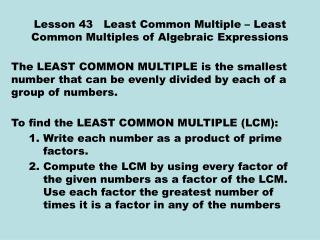DownloadDownload PresentationLesson 43 Least Common Multiple – Least Common Multiples of Algebraic Expressions

# Lesson 43 Least Common Multiple – Least Common Multiples of Algebraic Expressions

Download Presentation## Lesson 43 Least Common Multiple – Least Common Multiples of Algebraic Expressions

- - - - - - - - - - - - - - - - - - - - - - - - - - - E N D - - - - - - - - - - - - - - - - - - - - - - - - - - -
##### Presentation Transcript

1. Lesson 43 Least Common Multiple – Least Common Multiples of Algebraic Expressions The LEAST COMMON MULTIPLE is the smallest number that can be evenly divided by each of a group of numbers. To find the LEAST COMMON MULTIPLE (LCM): 1. Write each number as a product of prime factors. 2. Compute the LCM by using every factor of the given numbers as a factor of the LCM. Use each factor the greatest number of times it is a factor in any of the numbers

2. Examples Ex. 43.1 Find the LCM of 8, 15, 100. The prime factors of 8 are 2 x 2 x 2 The `prime factors of 15 are 3 x 5 The prime factors of 100 are 2 x 2 x 5 x 5 The number 2 is a factor. It will appear 3 times in the LCM. The number 3 is a factor. It will appear 1 time in the LCM. The number 5 is a factor. It will appear 2 times in the LCM. The LCM is: 2 x 2 x 2 x 3 x5 x 5 = 600

3. Lesson 43 Continued Ex 43.2 Find the LCM of 30, 75, and 80. Ex 43.3 Find the LCM of 560, 588, and 1250.

4. LCM Of Algebraic Expressions Ex 43.4 Find the LCM of 15a2b and 10ab3. 15a2b = 3 x 5 x a x a x b 10ab3 = 2 x 5x a x b x b x b Ex 43.5 Find the LCM of 4x2m and 6x3m. Ex 43.6 Find the LCM of 12x2m2 and 14x3am4. On Line Tutorial